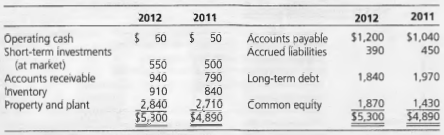### Create an Account

Home / Questions / Comprehensive Analysis and Valuation Hard This exercise comes in two parts Part I involves...

# Comprehensive Analysis and Valuation Hard This exercise comes in two parts Part I involves an analysis of a set of financial statements and Part II involves forecasting and valuation based on those

Comprehensive Analysis and Valuation (Hard) This exercise comes in two parts. Part I involves an analysis of a set of financial statements and Part II involves forecasting and valuation based on those financial statements. Part I: Analysis The following is a comparative balance sheet for a firm (in millions of dollars):The following is the statement of common shareholders&#39; equity:The firm&#39;s income tax rate is 35 percent. The firm reported \$15 million in interest income and \$98 million in interest expense for 2012. Sales revenue was \$3,726 million.

a. Calculate the loss to shareholders from the exercise of employee stock options.

b. The shares repurchased were in settlement of a forward purchase agreement. The market price of the shares at the time of the repurchase was \$25 each. What was the effect of this transaction on the income for the shareholders?

c. Prepare a comprehensive income statement that distinguishes after-tax operating income from financing income and expense. Include gains or losses from the transactions in parts (a) and (b ).

d. Prepare a reformulated comparative balance sheet that distinguishes assets and liabilities employed in operations from those employed in financing activities. Calculate the firm&#39;s financial leverage and operating liability leverage at the end of2012.

e. Calculate free cash flow for 2012.

Part II: Forecasting and Valuation Use a cost of capital for operations of9 percent. Sales revenue is forecasted to grow at a 6 percent rate per year in the future, on a constant asset turnover of 1.25. Operating profit margins of 14 percent are expected to be earned each year.

a. Forecast return on net operating assets (RNOA) for 2013.

b. Forecast residual operating income for 2013.

c. Value the shareholders&#39; equity at the end of the 2012 fiscal year using residual income methods.

d. Forecast abnormal growth in operating income for 2014.

e. Value the shareholders&#39; equity at the end of 2012 using abnormal earnings growth methods.

f. After reading the stock compensation footnote for this firm, you note that there are employee stock options on 28 million shares outstanding at the end of 2012. These options vest in 2014 and after. A modified Black-Scholes valuation of these options is \$15 each. How does this information change your valuation?

g. Forecast (net) comprehensive income for 2013.

May 25 2020 View more View LessSubscribe To Get Solution